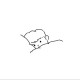# THUWC2020 游记

## Day 1

### T1

• 有 $0 \sim n$ 共 $n + 1$ 个具有 $k$ 维属性的物品。
• 一开始手上拿着第 $0$ 个物品，然后依次考虑物品 $1 \sim n$，若物品 $i$ 的第 $p_i$ 维属性要比当前手上拿着的物品的第 $p_i$ 维属性大，则放下手中的物品拿起第 $i$ 个物品。
• 现在，物品 $1 \sim n$ 都已经确定了，$q$ 次询问当确定第 $0$ 个物品时，最终剩下的物品是哪个。
• $n,q \le 10^5$，$k \le 20$。

### T2

• 给定一张 $n$ 个点 $m$ 条边的有向图，点的编号为 $1 \sim n$，边的编号为 $1 \sim m$，每条边有一个经过次数的上限 $w_i$。
• $q$ 次前面影响后面的询问，每次给定一个点 $x$ 和一个步数上限 $s$，求从 $x$ 开始每次走编号最小的出边，一直到走了 $s$ 步或没有出边可以走时停止，问停在哪个点上。
• $n,q \le 10^5$，$m \le 1.5 \times 10^5$，$w_i \le 10^{18}$，$s \le 10^9$。

### T3

• 给定一棵 $n$ 个点的树，$m$ 次询问只考虑编号在 $l \sim r$ 之间的点有多少个极大 $X$ 连通块。
• $X$ 连通块：对于一个点集 $S$，若对于任意 $i,j \in S$，都存在一个点序列，满足序列的每一项都属于 $S$，序列的首项为 $i$ 末项为 $j$，且相邻两项在树上的距离均 $\le X$，则称 $S$ 是一个 $X$ 连通块。
• $n \le 3 \times 10^5$，$m \le 6 \times 10^5$。

10 min 打完，不想拍，扔了，开 T2。

「某科学的动态仙人掌」，这什么玩意啊。

4h 了，果断放弃，扔了。

T3 似乎是 lxl 题？毒瘤！

## Day 2

### T1

• 有 $n$ 个函数，第 $i$ 个函数形如 $f_i(x) = a_i|x| + b_ix + c_i$。
• 你一开始有一个整数 $s$，你要按照某个 $1 \sim n$ 的排列的顺序经过这 $n$ 个函数。
• 当经过第 $i$ 个函数时，$s$ 要变成 $f_i(s)$。
• 求最终 $s$ 的最大值。
• $n,s,|a_i|,|b_i|,|c_i| \le 15$，可以使用 __int128

### T2

• 给定一棵 $n$ 个点的以 $1$ 为根的叶向树。
• 除了 $n-1$ 条有向树边之外，还有若干条从祖先到孩子的有向非树边，一共有 $m$ 条边。
• 有 $q$ 次询问，每次询问给定树上一条从祖先到孩子的路径 $a \to b$，需要求出去掉 $a \to b$ 上的所有树边后 $b$ 的子树中有多少个点无法从根到达。
• $n,q \le 10^5$，$m \le 1.5 \times 10^5$，保证无重边。

### T3

• 给定一棵 $n$ 个点的树，每个点有一个点权 $p_i$，点权互不相同且 $\in [1,n]$。
• 有 $m$ 次询问，每次询问给定两个节点 $u,v$ 和一个参数 $k$，设树上 $u \to v$ 的路径经过的所有点的点权为 $t_{1 \dots l}$，求有多少个序列经过 $k$ 轮冒泡排序后会变成 $t$。
• $n,m \le 5 \times 10^5$，答案对 $998244353$ 取模。

T1 的数据范围看起来很状压的样子，然后很 naive 的以为维护最大值就好了。

T3 瞪了半天也只会 5pts，15min 打完。

15min 数据分治交上去，过了但是一定会 fst，但也没办法了。

1.5h 调完大模拟拿到 40pts。

1h 搞完 T2 的前 6 个点，+40pts。

## Day 3

1. 我是从高一开始学 OI 的，所以现在还很菜。
2. 我创办了 X Round，办了四场，有 7.5k+ 人次参赛，也有集训队爷赏脸来打，但是这不是重点，重点是团队里其他人都是 THU 爷，就我不是，所以，嗯，THU 你们懂的。
3. 这是我的倒数第三次机会，还有一次 Summer Camp 和一次 NOI，但是要跟一群高中之前开始学 OI 的神仙拼还是很难。

## Day 4

4条评论
•Studying Father

2019年12月31日 上午12:44

我再一无所有，也还有小粉兔呢（

这个理由可以（

1.xht37

2020年1月6日 下午11:36

嘻嘻

•ezoixx130

2020年2月21日 上午10:14

%xht37 顺便问下Day3的材料哪来的啊

1.xht37

2020年2月21日 下午1:25

忘了…感觉好多地方都有